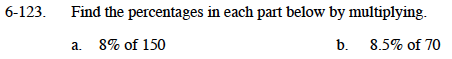### Home > MC1 > Chapter 6 > Lesson 6.3.1 > Problem6-123

6-123.
1. . Find the percentages in each part below by multiplying. Homework Help ✎

1. 8% of 150

2. 8.5% of 70Convert 8% to a fraction or a decimal and then multiply by 150.

8% as a decimal is 0.08.

8.5% as a decimal is 0.085.

5.95